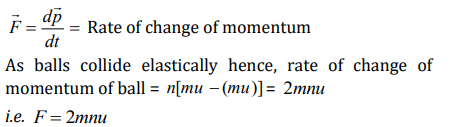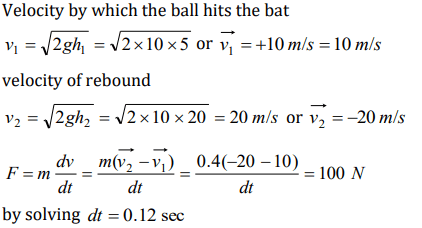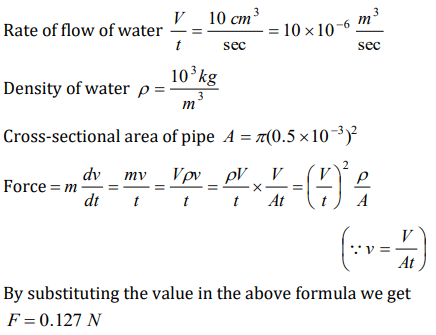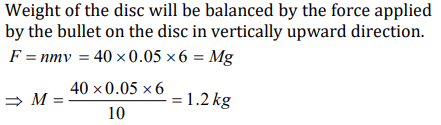## Laws of Motion Questions and Answers Part-11

1. A body of mass 8kg is moved by a force , $F=3xN$  ,where x is the distance covered. Initial position is $x=2m$  and the final position is $x=10m$  . The initial speed is 0. 0 m/s . The final speed is
a) 6 m/s
b) 12 m/s
c) 18 m/s
d) 14 m/s

Explanation:2. The linear momentum p of a body moving in one dimension varies with time according to the equation $P=a+bt^{2}$  , where a and b are positive constants. The net force acting on the body is
a) Proportional to $t^{2}$
b) A constant
c) Proportional to t
d) Inversely proportional to t

Explanation:3. A ball of mass 0.5 kg moving with a velocity of 2 m/sec strikes a wall normally and bounces back with the same speed. If the time of contact between the ball and the wall is one millisecond, the average force exerted by the wall on the ball is
a) 2000 N
b) 1000 N
c) 5000 N
d) 125 N

Explanation:4. A particle moves in the xy-plane under the action of a force F such that the components of its linear momentum p at any time t are $p_{x}=2\cos t$  , $p_{y}=2\sin t$  . The angle between F and p at time t is
a) $90^{\circ}$
b) $0^{\circ}$
c) $180^{\circ}$
d) $30^{\circ}$

Explanation:5. n small balls each of mass m impinge elastically each second on a surface with velocity u. The force experienced by the surface will be
a) mnu
b) 2 mnu
c) 2 mnu
d) $\frac{1}{2} mnu$

Explanation:6. A ball of mass 400 gm is dropped from a height of 5m. A boy on the ground hits the ball vertically upwards with a bat with an average force of 100 newton so that it attains a vertical height of 20 m. The time for which the ball remains in contact with the bat is $\left[g=10 m\diagup s^{2}\right]$
a) 0.12s
b) 0.08 s
c) 0.04 s
d) 12 s

Explanation:7. A gun of mass 10 kg fires 4 bullets per second. The mass of each bullet is 20 g and the velocity of the bullet when it leaves the gun is $300 ms^{-1}$  .The force required to hold the gun while firing is
a) 6 N
b) 8 N
c) 24 N
d) 240 N

Explanation: Rate of change of momentum of the bullet in forward direction = Force required to hold the gun. F = nmv = 4 * 20 * 10-3 * 300 = 24 N

8. A gardner waters the plants by a pipe of diameter 1mm. The water comes out at the rate or $10 cm^{3}\diagup sec$   . The reactionary force exerted on the hand of the gardner is
a) Zero
b) $1.27\times10 ^{-2}N$
c) $1.27\times10 ^{-4}N$
d) 0.127 N

Explanation:9. A solid disc of mass M is just held in air horizontally by throwing 40 stones per sec vertically upwards to strike the disc each with a velocity $6 m s^{-1}$ . If the mass of each stone is 0.05 kg. what is the mass of the disc $\left(g=10 m\diagup s^{2}\right)$
a) 1.2kg
b) 0.5kg
c) 20kg
d) 3kg